## How to print long column on one page in Excel?

Supposing you have a long list of data in a column, maybe 200 rows, and now you need to print them, but when you print, it uses about 5 page paper, with only one column at the left side, and much white space on the right side. In word, you can column, but Excel doesn't have this function. How do you print the long list data on one page to save the paper?

Print long column on one page with formula

Print long column on one page with VBA code

Print long column on one page with Kutools for Excel

####Print long column on one page with formula

Here I can introduce you a long formula to solve this problem, please do as this:

1. In a new worksheet of your active workbook, enter the formula =IF(OFFSET(Sheet1!\$A\$1,(COLUMN()-1)*45+ROW()-1,0)="","",OFFSET(Sheet1!\$A\$1,(COLUMN()-1)*45+ROW()-1,0)) into the cell A1.

Notes: Sheet1 is the worksheet which has the long list that you want to column.

45 is the row number that you want to list the data in a column.

They are all variables, and you can change them as your need.

2. Then select cell A1, and drag the fill handle down to row 45, and then go on dragging the fill handle across to right until the data has been displayed. And the long column has been divided into several columns to fit on one page in a new worksheet. See screenshot:####Print long column on one page with VBA code

The following VBA code also can help you divide a long list of data into several columns, so that you can print the data and save paper.

1. Hold down the ALT + F11 keys, and it opens the Microsoft Visual Basic for Applications window.

2. Click Insert > Module, and paste the following code in the Module Window.

``````Sub SingleToMultiColumn()
Dim rng As Range
Dim iCols As Integer
Dim lRows As Long
Dim iCol As Integer
Dim lRow As Long
Dim lRowSource As Long
Dim x As Long
Dim wks As Worksheet
Set rng = Application.InputBox _
(prompt:="Select the range to convert", _
Type:=8)
iCols = InputBox("How many columns do you want?")
lRowSource = rng.Rows.Count
lRows = lRowSource / iCols
If lRows * iCols <> lRowSource Then lRows = lRows + 1
lRow = 1
x = 1
For iCol = 1 To iCols
Do While x <= lRows And lRow <= lRowSource
Cells(x, iCol) = rng.Cells(lRow, 1)
x = x + 1
lRow = lRow + 1
Loop
x = 1
Next
End Sub
``````

3. Then press the F5 key to run this code, and select the list data that you want to divide in the popped up dialog box, see screenshot: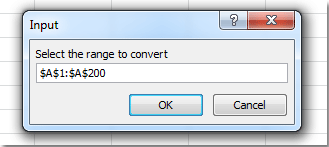4. Click OK, and enter the number of columns you want to divide. See screenshot: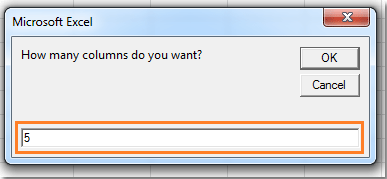5. And click OK, the long column has been divided into five columns in a new worksheet. See screenshots: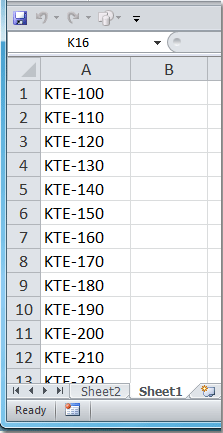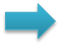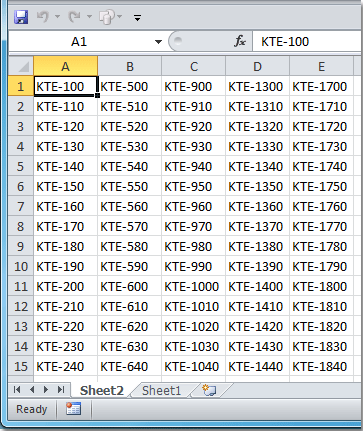####Print long column on one page with Kutools for Excel

Both the long formula and the VBA code may be difficult for you, here I can introduce you an easy and convenient method to solve this problem. Kutools for Excel’s Print Multiple Columns function can help you quickly divide the long column into several columns so that you can print them reasonably.

Kutools for Excel includes more than 300 handy Excel tools. Free to try with no limitation in 30 days. Get it Now.

When you have installed Kutools for Excel, you can do as following steps:

1. Click Enterprise > Print Multiple Columns, see screenshot: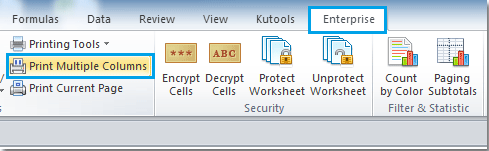2. In the Print Multiple Columns dialog box:

• Click the first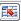button to select the title of the range, and then click the secondbutton to select the column that you want to divide.
• Then specify the number of rows you want to put per page from Rows per printed page, and enter the number of columns that you want in one page under Number of segments. See screenshot: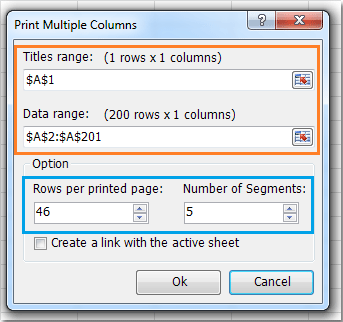3. Then click OK, the long list data has been divided into five columns in a page. See screenshots: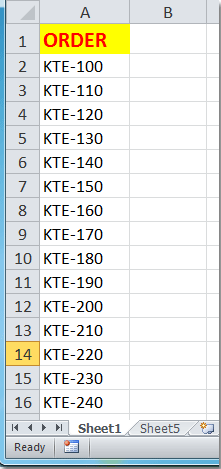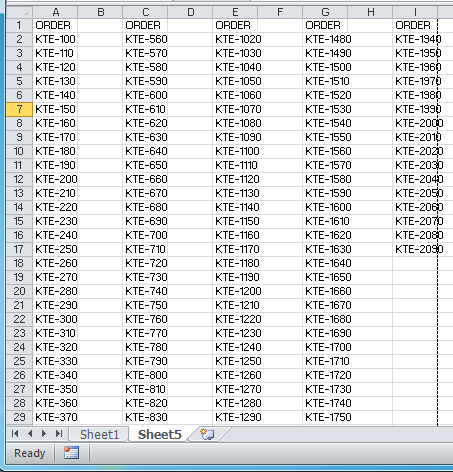Notes:

1. The title will be added before every column.

2. If you check Create a link with the active sheet option, the new worksheet data can be linked to the source data.

### Best Office Productivity Tools

 Popular Features: Find, Highlight or Identify Duplicates   |  Delete Blank Rows   |  Combine Columns or Cells without Losing Data   |   Round without Formula ... Super Lookup: Multiple Criteria VLookup  |   Multiple Value VLookup  |   VLookup Across Multiple Sheets   |   Fuzzy Lookup .... Advanced Drop-down List: Quickly Create Drop Down List   |  Dependent Drop Down List   |  Multi-select Drop Down List .... Column Manager: Add a Specific Number of Columns  |  Move Columns  |  Toggle Visibility Status of Hidden Columns  |  Compare Ranges & Columns ... Featured Features: Grid Focus   |  Design View   |   Big Formula Bar   |  Workbook & Sheet Manager   |  Resource Library (Auto Text)   |  Date Picker   |  Combine Worksheets   |  Encrypt/Decrypt Cells   |  Send Emails by List   |  Super Filter   |   Special Filter (filter bold/italic/strikethrough...) ... Top 15 Toolsets:  12 Text Tools (Add Text, Remove Characters, ...)   |   50+ Chart Types (Gantt Chart, ...)   |   40+ Practical Formulas (Calculate age based on birthday, ...)   |   19 Insertion Tools (Insert QR Code, Insert Picture from Path, ...)   |   12 Conversion Tools (Numbers to Words, Currency Conversion, ...)   |   7 Merge & Split Tools (Advanced Combine Rows, Split Cells, ...)   |   ... and more

Supercharge Your Excel Skills with Kutools for Excel, and Experience Efficiency Like Never Before. Kutools for Excel Offers Over 300 Advanced Features to Boost Productivity and Save Time.  Click Here to Get The Feature You Need The Most...#### Office Tab Brings Tabbed interface to Office, and Make Your Work Much Easier

• Enable tabbed editing and reading in Word, Excel, PowerPoint, Publisher, Access, Visio and Project.
• Open and create multiple documents in new tabs of the same window, rather than in new windows.
• Increases your productivity by 50%, and reduces hundreds of mouse clicks for you every day!Rated 4.5 out of 5 · 1 ratings
This comment was minimized by the moderator on the site
I have a column of 10,000 unique numbers, all sorted. The VBA code provides a table, but the Columns are sorted individually.
I would prefer that each page is sorted numerically so easy to follow the data page by page. Any suggestions most welcome.
Keith
This comment was minimized by the moderator on the site
Hello, Paterson,
Maybe the following VBA code can do you a favor:
``````Sub SingleToMultiColumn()
Dim xRng As Range
Dim xCount As Integer
Dim xICols As Integer
Dim xLRows As Long
Dim xICol As Integer
Dim xLRow As Long
Dim xLRowSource As Long
Dim xRowNum As Long
Dim xFCount, xFNum, xFNum_R, xFNum_C As Long
Dim x As Long
Dim xWst As Worksheet
Dim xJ As Integer

Set xRng = Application.InputBox(prompt:="Select the range to convert", Type:=8)
xICols = InputBox("How many columns do you want?")
xRowNum = InputBox("How many rows do you want in a page?")
xCount = xRng.Count
xFCount = Fix(xCount / (xICols * xRowNum))
If xICols * xRowNum * xFCount < xCount Then xFCount = xFCount + 1
xJ = 0
Application.ScreenUpdating = False
For xFNum = 1 To xFCount
If xJ = xCount Then Exit For
For xFNum_C = 1 To xICols
If xJ = xCount Then Exit For
For xFNum_R = 1 To xRowNum
If xJ = xCount Then Exit For
xWst.Cells((xFNum - 1) * xRowNum + xFNum_R, xFNum_C) = xRng.Item(xJ + 1)
xJ = xJ + 1
Next
Next
Next
Application.ScreenUpdating = True
End Sub

``````

This comment was minimized by the moderator on the site
I have a column of 10,000 numbers and the VBA macro will provide a table on several pages. However, I would prefer that each page is sorted numerically rather than the column. Any help appreciated.
This comment was minimized by the moderator on the site
Or you can just copy the table, and paste it in a word document, with as many columns you want to have.
This comment was minimized by the moderator on the site
Thank you! The formula worked seamlessly!
This comment was minimized by the moderator on the site
You can use a simple formula =OFFSET(Sheet1!\$A\$1,(ROW()-2)*5+COLUMN(),0) to let the data flow horizontally in 5 columns. Then you can select Page size, print first row on each page, etc. Simple, neat, no VBA
This comment was minimized by the moderator on the site
where does this formula go? I am trying to print multiple rows with several columns on one page in a multiple row layout. Similar to this:

john smith week 1
john smith week 2
john smith totals

I have over 100 rows with up to IJ
This comment was minimized by the moderator on the site
Is there a way to modify this formula to work with 3 columns instead of 1?
This comment was minimized by the moderator on the site
Modern Excel (e.g. 365) can use this for single column:
=WRAPCOLS(Sheet1!\$A\$1:\$A\$50;45;"")

Similarly, if you want the column spread horizontally use:
=WRAPROWS(Sheet1!\$A\$1:\$A\$50;3;"")

If you need multiple columns converted, I created this formula:
=LET(SOURCE;Sheet1!\$A\$1;R;45;C;3;X;MOD(COLUMN()-1;C);NEW_REL_COL;INT((COLUMN()-1)/C);Y;(NEW_REL_COL)*R+ROW()-1;V;OFFSET(SOURCE;Y;X);IF(OR(V="";ROW()>R);"";V))

Update SOURCE (Sheet1!\$A\$1) to be the top-left corner of your source, the number behind R (45) for the rows; the number behind C (3) for the columns of your source.

e.g. for output of 2 columns with 30 rows start the formula with =LET(SOURCE;Sheet1!\$A\$1;R;30;C;2;

On modern Excel (e.g. 365) can use this Spill-Function for multicolumn with an added empty column in between:

=LET(R;45;C;3;EMPTY_COL;TRUE;
E;IF(EMPTY_COL;1;0);ANZ;COUNTA(Sheet1!\$A:\$A);MY;R;MX;CEILING.MATH(ANZ/MY;1)*(C+E);
MAKEARRAY(MY;MX;LAMBDA(ru;co;
LET(X;MOD(co-1;(C+E));NEW_REL_COL;INT((co-1)/(C+E));Y;(NEW_REL_COL)*R+ru-1;
IF(OR(AND(EMPTY_COL;X=(C+E-1));Y>=ANZ);"";OFFSET(Sheet1!\$A\$1;Y;X))
))))

Needs to be filled only in one cell and fills out down and right.
Use R and C like above. Fill the value EMPTY_COL with true or false if you want an empty column to be added between the repetitions.
Rated 4.5 out of 5
This comment was minimized by the moderator on the site
I would be interested in a solution like this as well.
This comment was minimized by the moderator on the site
Dear Sir Thanks for this work, This VBA Code is very helpful for my work, I am glad to complete my task in seconds. Thank You. ....
This comment was minimized by the moderator on the site
After searching the internet for hours, I stumbled across this. Its exactly what I was looking for. Nice job and thanks for taking the time out for this.
This comment was minimized by the moderator on the site
Thank you for this information! It was very helpful and easy to use - even for somebody lacking strong computer skills (I used the first method)
This comment was minimized by the moderator on the site
Hello, I have a question related to the second option "Print long column on one page with VBA code". If i have more than one column in range how can I use the same code. Let say the range of "\$A\$2:\$C\$118" how can i do it work. Thanks.
There are no comments posted here yet